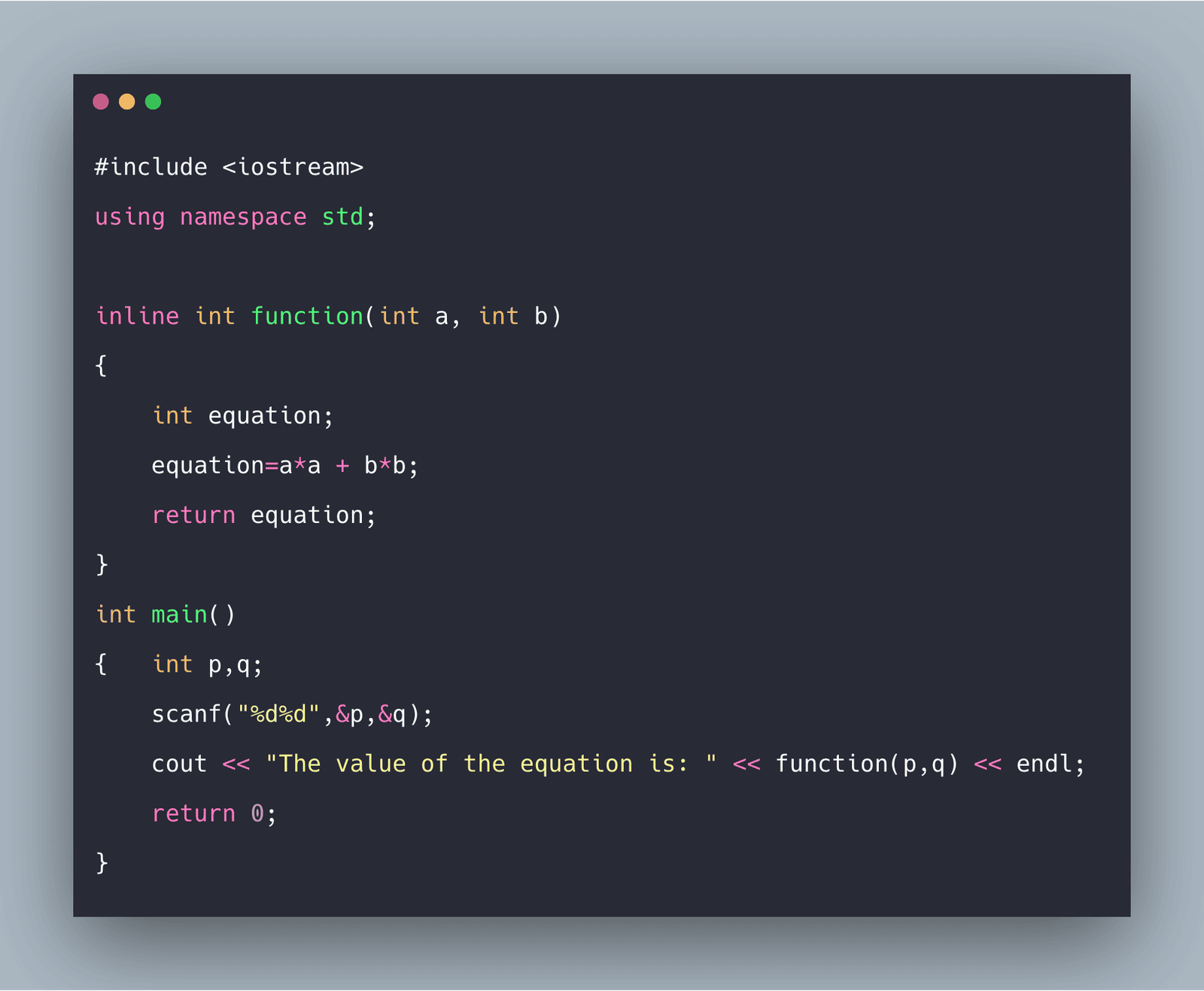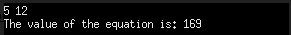# C++ Inline Functions: The Complete Guide

0
7Inline functions are a great way to define small functions whose execution time is less than the time it takes to transit the control from the called function to the calling function.

## C++ Inline Function

The inline function in C++ substitutes the function code that is to be executed in the main program code itself in the place where it is called. So it automatically eliminates the time it takes to switch the control from the called function to the main program, making the whole program much more efficient.

### Syntax

See the following syntax.

```inline return_valuetype function_name()
{
//function definition;
}
```

### Example

See the following code example.

```inline int add(int a, int b)
{
int sum=0;
sum=a + b;
return sum;
}```

## Problem with Small Functions

When we use small functions for pretty small tasks in our program, they take almost negligible time to execute the commands.

Instead, it takes more time to take the execution control from the function call statement in the main() function to the function definition and get it back to the next statement after the function call statement.

But functions are necessary to make the program modular. So the workaround for this is the inline functions. They eliminate the time compiler takes to move the execution control.

They do so by placing the function definition from where it is defined to the point where the function call statement is made, in line with the main program like the general code during the compilation time.

## Program to declare inline functions

See the following program.

```#include <iostream>
using namespace std;

inline int function(int a, int b)
{
int equation;
equation=a*a + b*b;
return equation;
}
int main()
{   int p,q;
scanf("%d%d",&p,&q);
cout << "The value of the equation is: " << function(p,q) << endl;
return 0;
}```

See the following output.## Advantages of Using Inline Functions

### Saves Push/Pop operations

Functions have separate copies of variables for them with local scope, and they need to be passed/ returned to the main function after the execution of the function. It saves the system’s time to first the values in local variables and then pass it over to the global ones. Instead of inline functions, since they are a part of the main program, the values are directly stored in the global variables.

### Beneficial for Embedded systems

Inline functions are great to run embedded system programs. However, they hold too little memory with limited hardware to store software files. Inline functions make the compiled file (ready to be executed) small in size compared to those made using standard function definition.

### It makes the program more optimized.

When the compiler compiles the program, it automatically improves the flow inside the main() function to make the program efficient. But this is not the case with the functions defined inside the main() function. Instead, using the inline function, we transfer the function definition to the main() function as it is written initially there. Hence, the compiler improves that piece of code and makes the program more efficient.

## Disadvantages of Using Inline Functions

One major disadvantage of using inline functions is the number of extra memory registers at once. With standard function calls, the memory registers used for the function definition get freed up when it returns to the main() function.

Since the inline function is executed with the main() function itself, the registers are used simultaneously. And if the amount of variables declared is more than the registers available, it takes a significant portion of time for the program to get executed.

### Increases the size of the executable file

The thing with inline functions is that it increases code redundancy. When the compiler compiles the program, the inline function definition is placed in the main() program anywhere it is called.

So if you have made too many inline function calls, the function definition will be introduced in the main() program at many places, thereby increasing the size of the executable file and decreasing the efficiency of the program.

### Increases the compilation time

If any change has to be made in the program, it must be recompiled. With standard function definitions, the changes in the function definitions are compiled only once where they are declared, but with inline functions.

Since the code is mentioned many times in the main() function, it is compiled every time in the program. Therefore, various compilations of the same definition add up and increase the compilation time of the program.

That’s it for this tutorial.

This site uses Akismet to reduce spam. Learn how your comment data is processed.